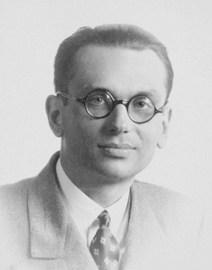# Gödel’s Second Incompleteness Theorem Explained in Words of One SyllableGeorge Boolos in Mind (1994) [h/t: Dan Balis]:

First of all, when I say “proved”, what I will mean is “proved with the aid of the whole of math”. Now then: two plus two is four, as you well know. And, of course, it can be proved that two plus two is four (proved, that is, with the aid of the whole of math, as I said, though in the case of two plus two, of course we do not need the whole of math to prove that it is four). And, as may not be quite so clear, it can be proved that it can be proved that two plus two is four, as well. And it can be proved that it can be proved that it can be proved that two plus two is four. And so on. In fact, if a claim can be proved, then it can be proved that the claim can be proved. And that too can be proved.

Now, two plus two is not five. And it can be proved that two plus two is not five. And it can be proved that it can be proved that two plus two is not five, and so on.

Thus: it can be proved that two plus two is not five. Can it be proved as well that two plus two is five?

More here.